# MATLAB Syntax

• Last Updated : 05 Oct, 2021

Writing code in the MATLAB environment is quite simple. We do not need to include any libraries/header files, we can directly start writing commands in the command window of the Editor. Usually, we write small and easily executable programs in the Command Window and larger programs with multiple lines and functions in the Editor.

Now, we will see the syntax of a MATLAB program. Let us begin with the very basic code to display ‘Hello World’ as the output in the command window:

Example:

## Matlab

 `% A MATLAB program illustrate``% disp function``disp(``"Hello World"``)`

Here, disp() is a function used to display the desired value as the output.

Output: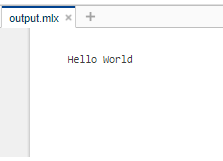Likewise, we can perform any basic operation in the command window. Let’s have a look at a few of them.

Example :

## Matlab

 `% Adding two numbers in the``% MATLAB Command Window``15 + 25`

Output :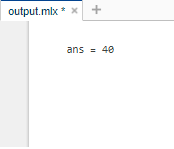In the above output, ‘ans’ is a default variable in MATLAB that stores the value of the most recent output. This variable is only created when output is generated without any specific argument to it.

Example:

## Matlab

 `% MATLAB code for multiplying two numbers``% in MATLAB Command Window``20 * 5`

Output :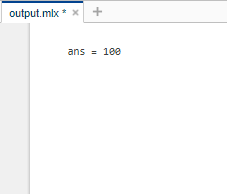Thus, we can perform various mathematical operations in the MATLAB command window.  The following table summarizes the various operations along with their syntax that can be performed in MATLAB:

## Declaring Variables in MATLAB

Declaring Variables in MATLAB is fairly simple. We just need to write a valid name for the variable followed by an equal sign (‘=’). The naming of variables is discussed further in this article. In MATLAB, we need not explicitly mention the type of variable while declaring it,

a=7                                %declares an integer variable and assigns it the value 7

b=9.81                            %declares a float (decimal) variable and assigns it the value 9.81

c = [1,2,3]                       %declares an array and assigns it the values 1, 2 and 3

d=a = [1,2,3;4,5,6;7,8,9]  %declares a 2-D array and assigns it the values 1,2,3,4,5,6,7,8 and9

## Naming Variables in MATLAB

The rules for naming a variable in MATLAB are as follows:

• A variable name must begin with a letter which may be followed by letters, digits, or underscores (no other special character allowed).
• MATLAB is case-sensitive which means that upper and lower case letters are considered different. (‘GeeksForGeeks’ and ‘geeksforgeeks’ are considered separate variable names)
• Avoid giving variables the same name as that of existing MATLAB functions.

## Use of semicolon in MATLAB

Unlike many programming languages, every statement/expression in MATLAB need not necessarily end with a semicolon. However, in MATLAB, a semicolon is used at the end of a statement to restrict the result of the statement from being displayed in the output.

Let us understand this with a few examples:

Example:

## Matlab

 `% MATLAB code to assign values``% to two variables and display``% their sum (without the use of``% any semicolon)``a=2``b=3``c=a+b`

Output: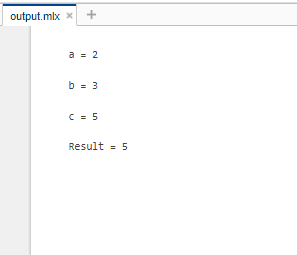In the above code, although we didn’t write any explicit statement to print the value stored in the variable a, b and c, yet the values of a, b and c is displayed as the output by default.

Example:

## Matlab

 `% MATLAB code to assign values``% to two variables and display``% their sum (with the use of``% semicolon)``a = 2;``b = 3;``c = a+b`

Output: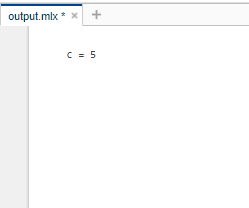We can observe that this time the output of the statements ending with a semicolon (a=2; and b=3;) are not displayed as the output. Hence, a semicolon can be used in MATLAB to restrict the result of the statement from being displayed in the output.

Note: A semicolon does not restrict the output from being displayed when put at the end of a statement that is specifically meant to print output.

Example:

## Matlab

 `% MATLAB code to disp function %``% (with the use of semicolon) %` `disp(``'Learning with Geeks for Geeks'``);`

Output: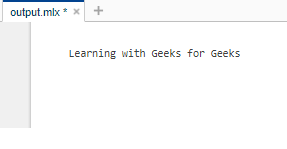Adding comments to code is always considered a good practice. Comments are statements or annotations written within the source code to make the code easier for humans to understand. Comments are simply ignored at the time of compilation/execution.

MATLAB provides two types of comments:

Single-Line Comments: They are denoted by ‘%’ (percentage sign). It implies that the comment is applied to a single line only which means that everything following ‘%’ in a line is a comment and thus not executed.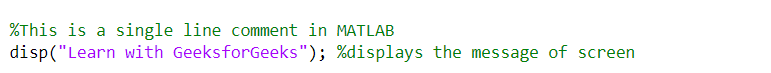Multi-Line Comments: They are denoted by ‘%{‘ (percentage sign and opening curly bracket) and ‘%}’ (percentage sign and closing curly bracket). Everything is written between ‘%{‘ and ‘%}’ is a comment and thus not executed.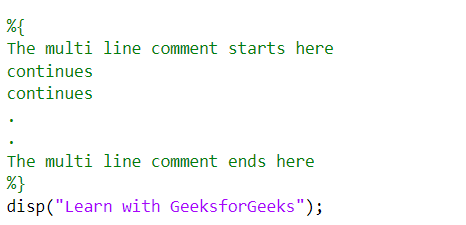## Saving work in MATLAB

In order to save a MATLAB script, go to the editor tab and click ‘save as’, and then provide your script with an appropriate name. The scripts written in MATLAB are stored with the ‘.m’ extension.

My Personal Notes arrow_drop_up